# Matrices and Operations — Linear Algebra for QC

## Last week we talked about vectors, now we are going to discuss matrices and the operations they perform on vectors.

This is the second article of our series talking about the fundamentals of linear algebra and everything you need to know about it in order to work with it in Quantum Computing. You can find the first article, which talks about vectors and scalars, here.

Okay, now that we have a solid understanding of what vectors and scalars are, we can begin to explore matrices. While they have a great variety of functions and applications throughout linear algebra, for our purposes in quantum computing, matrices can be purely thought of as operations acting on vectors.

Let’s look at a simple matrix.

A fundamental property of matrices is their shape, which is generally represented in the form “# of rows x # of columns”. The matrix above has shape 2x2, which means it is a square matrix (it has the same number of rows and columns). For quantum computing — at least starting off — you are going to use what are called Hermitian matrices, which (among other things) means that the matrix is square.

Because of this, we are only going to focus on square matrices in this article, but most concepts can be easily extended to rectangular matrices (those where the number of rows is not equal to the number of columns) and there are plenty of resources to study them, i.e. MIT’s OCW Linear Algebra course.

Another important feature of matrices is that they are composed of various elements. In the example above, you can clearly see that it has 4 elements: 1, 2, 4, and 3. But matrices can get pretty large and we need a way too label each element. Take a look at the labeling of the elements of the matrix A and see if you find a pattern.

You may have notice that the sub-index of each element corresponds to the location of that element in the matrix. For example, the element at row 2 and column 1 is 4, which is denoted as “a” with sub-index (21). It may be helpful to think of the indices as two separate numbers (“one two”, “one one”, etc.) rather than a single number (“twelve”, “eleven”, etc.). This way, you can easily identify the row and column the element refers to.

Now that we have a basic overview of what is a matrix, we can see what we can do with them.

Matrix addition is pretty straightforward, you just add the corresponding components of each matrix to get the result. Let’s say that we have two matrices, A and B.

Now, we want to find C, which is the addition of A and B.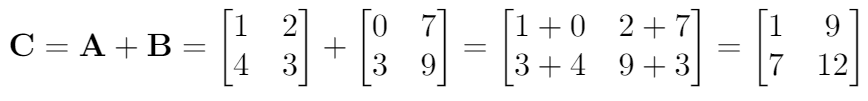Matrix C, defined to be the addition of A and B

As you can see, matrix addition is not complicated at all, you just need to keep track of which element you are adding and make sure you don’t mix up elements at different positions.

## Matrix multiplication

Matrix multiplication is a bit harder to understand at first, but it gets easier with a lot of practice. Before getting into this, make sure that you understand how to take the dot product of two vectors. If you don’t know what this is, I recommend you read this article I wrote and scroll down until you find the section on dot product.

Once you are familiar with the dot product, matrix multiplication is not that hard, since it is only composed of many dot products.

Let’s look at an example and then we will explain what goes on in matrix multiplication. First, we define two matrices.

Now, let’s see what multiplication looks like.

A lot is going on in this picture, but don’t worry, there is an easy pattern to follow. Let’s look at the element at position 11 in the resultant matrix. Try to see how matrices X and Y interact to get this element.

It’s simply the dot product of the first row of X with the first column of Y. Let me show you.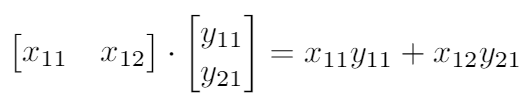Dot product of the first row of X with the first column of Y

You may be able to see the pattern from now on. The element at the position 12 in the resultant matrix is just the dot product of the first row of X with the second column of Y. Similarly, the element at position 21 is the dot product of the second row of X with the first column of Y, and the element at position 22 is the dot product of the second row of X with the second column of Y.

This pattern is all you need to remember to do matrix multiplication, and it can be easily extended to matrices with any shape — as long as the one at the left has the same number of columns as the number of rows in the one at the right.

Why is this? Before giving you the answer, try to multiply a 1x3 matrix with a 2x4 matrix. Why doesn’t this work? This has to do with the dot product- you cannot take the dot product of two vectors that don’t have the same number of elements — from this follows that matrices that don’t meet the requirement established in the last paragraph can’t be multiplied.

One more thing before moving on (which you may have already noticed) is that matrix multiplication is not commutative, which means that the order of the factors alters the result. For example, let’s multiply Y and X as defined above, in that order.

As you can see, this is not the same as XY. The difference again comes from the definition of dot product and matrix multiplication itself. If you want a more in depth review of matrix multiplication, I recommend watching the first half (or all of it!) of this lecture.

## Matrix-Vector Multiplication

As you may have guessed by now, vectors are actually just a simpler form of matrices! When we represent a vector, we put its components into a matrix with just one column, and each of its components taking up its own row. Let’s take some vector “v”, and represent it in both component and column form:

We can multiply this vector by any matrix as long as it has the same number of columns as this one has rows, in this case being 3. For an example, let’s multiply this vector by some matrix “U”:

The multiplication will work as follows: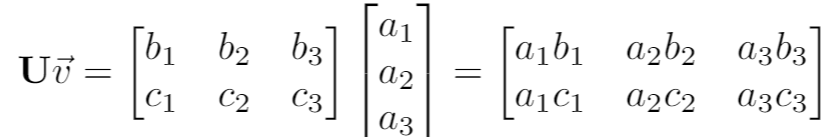We use the exact same process as described before

To give you a quick overview of how matrix multiplication works on quantum computing, let’s perform a very common operation — the quantum bit flip. Suppose we have a state described by the vector below.

To perform the quantum bit flip operation, we are going to apply the following matrix.

Hence, the operation will look as follows.

As you can see, our resultant state is flipped, exactly what we wanted! This demonstrates the power of linear algebra to represent various quantum gates, which act on qubits like matrices act on vectors.

## Bringing It Together - Hermitian Matrices And QC

Now that you know how to work with and manipulate vectors and matrices, it’s time to see how they apply in the field of quantum computing! The very necessity of these constructs and linear algebra as a whole in this field arises from the necessity to describe qubits and quantum states mathematically. We have entire articles devoted to explaining how exactly we represent and manipulate such quantum states using the math described using the linear algebra we’ve been learning thus far, and hopefully they give you a good insight as to how and why we need these tools.

We briefly introduced the notion of “Hermitian” matrices at the beginning of this article, and despite their fancy terminology, they are primarily just “square matrices”, those which have the same number of rows and columns, that are their own conjugate transpose- all this means is that the element at the position ij is now equal to the complex conjugate of the element at the position ji. And complex conjugate is when you invert the sign of the imaginary part of a complex number — thus, it has no effect in numbers with no imaginary part.

For example, take the following number.

Its complex conjugate, denoted by a bar top of the number, will be the following.

To illustrate this concept, let’s look at a matrix with some complex numbers as elements and take a look at what it’s complex conjugate — denoted by a dagger superscript — looks like.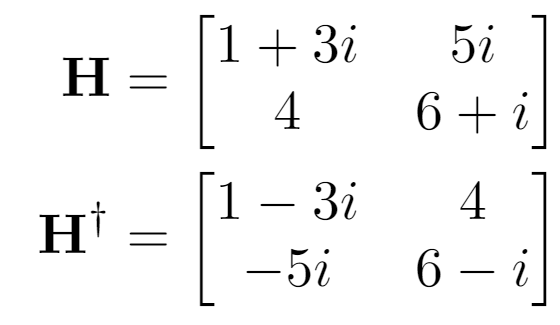A matrix H and its complex conjugate

As you may see, not only the sign for all imaginary parts changed, but also the rows and columns were exchanged — row 1 is now column 1 and row 2 is now column 2. This step is called transposing the matrix. And, as you may have already noticed, the matrix H is not Hermitian since it is not equal to its complex conjugate.

Because of their nature, these matrices exhibit many special properties (which we will go into more depth in the future). Quantum computing makes great use of a number of very specific Hermitian matrices, like the Pauli matrices, shown below: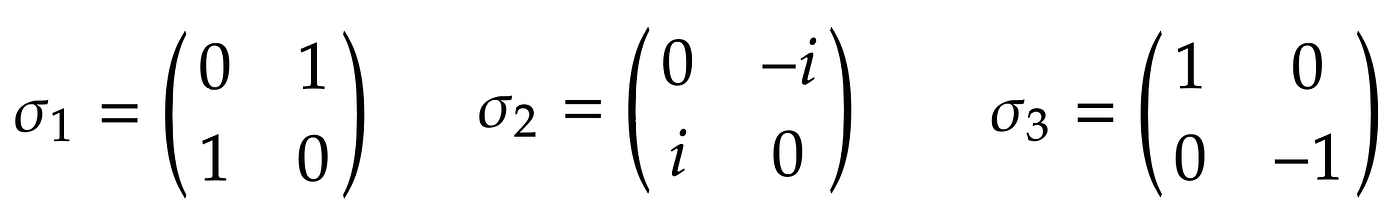Pauli matrices. Via Dirac for the Rest of Us.

Again, we review the application of these matrices in greater detail in the articles mentioned above, but we primarily use such constructs as operators. In quantum mechanics and QC, we like to represent certain manipulations and phenomena in the form of these Hermitian matrices which we refer to as operators, with which we can apply to quantum states in the form of vectors- using the exact matrix-vector multiplication processes shown above!

This concludes this in-depth review into just how matrices work and how we can do simple math with them. These constructs are absolutely necessary, not just in the field of QC but in sciences and technologies used around the world! Thank you for joining us today, and stay tuned as we at Quantum Untangled continue to explore the vast world of quantum phenomena to make quantum accessible to everyone!

--

--